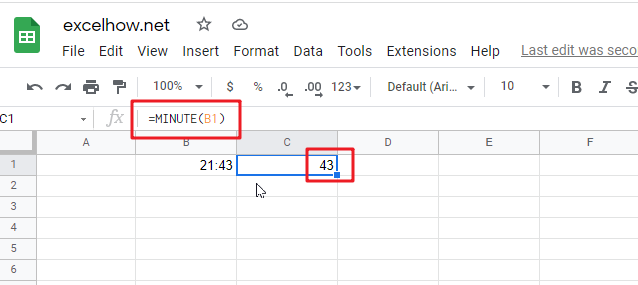This post will guide you how to use Google Sheets MINUTE function with syntax and examples.

Description

The Google Sheets MINUTE returns the minutes of a time value. And the minutes is an integer number from 0 to 59. So you can use the MINUTE function to extract the minute component of a given time value.

The MINUTE function can be used to extract the minute component of a given time as a number between 0-59 in google sheets. The purpose of this function is to get minute as a number from a time and its returned values is a number between 0 and 59.

For example, for a valid time value 10:29 AM, the MINUTE function will return 29. So you can pass the returned result into another formula.

The MINUTE function is a build-in function in Google Sheets and it is categorized as a Date function.

Syntax

The syntax of the MINUTE function is as below:

=MINUTE (time)

Where the MINUTE function arguments is:

• time -This is a required argument. A valid time value that contains the minute you want to extract. The times can be entered as a text strings within quotation marks (for example, “10:32 AM“), or typed as a decimal numbers, or the results of others formulas. It must be a cell reference that containing a date or time, or a function that returning a date/time type or a number.

Note:

• Time values are a portion of a date value and represented by a decimal number (for example, 12:00 PM is represented as 0.5, since it is half of a day).
• If the time is not a valid Excel time, it will return #VALUE! Error.
• Time argument can be supplied to the MINUTE function as a time text string or as a decimal numbers. If you wish to create a time value with separate hour, minute and second, you can use the TIME function.

The below examples will show you how to use google sheets MINUTE Function to return an integer number that representing the minute component of a given time.

#1 get minute portion of the time, enter the following formula in Cell C1.

=MINUTE(B1)Related Posts

This post will guide you how to use Google Sheets EXP function with syntax and examples. Description The Google Sheets EXP function returns e raised to the power of a number.  The constant e is equal to 2.71828182845904, and it ...

This post will guide you how to use Google Sheets FACT function with syntax and examples. Description The Google Sheets FACT function returns the factorial of a given number.  The factorial of a given number is equal to 1*2*3…* number. ...

This post will guide you how to use Google Sheets EVEN function with syntax and examples. Description The Google Sheets EVEN function rounds a given number up to the nearest even integer. You can use the EVEN function to return ...

This post will guide you how to use Google Sheets COUNTBLANK function with syntax and examples. Description The Google Sheets COUNTBLANK function use to count the number of empty cells in a range of cells. You can use the COUNTBLANK ...

This post will guide you how to use Google Sheets DEGREES function with syntax and examples. Description The Google Sheets DEGREES function converts radians into degrees. And it will return a numeric value. For examples, if you pass the PI() function into ...

This post will guide you how to use Google Sheets COSH function with syntax and examples. Description The Google Sheets COSH function returns the hyperbolic cosine of a given angle. The purpose of this function is used to get hyperbolic ...

This post will guide you how to use Google Sheets COS function with syntax and examples. Description The Google Sheets COS function returns the cosine of a given angle. If you want to supply an angle to COS function in ...